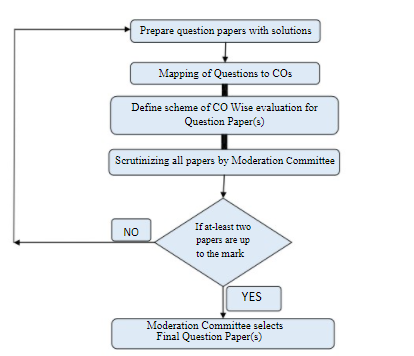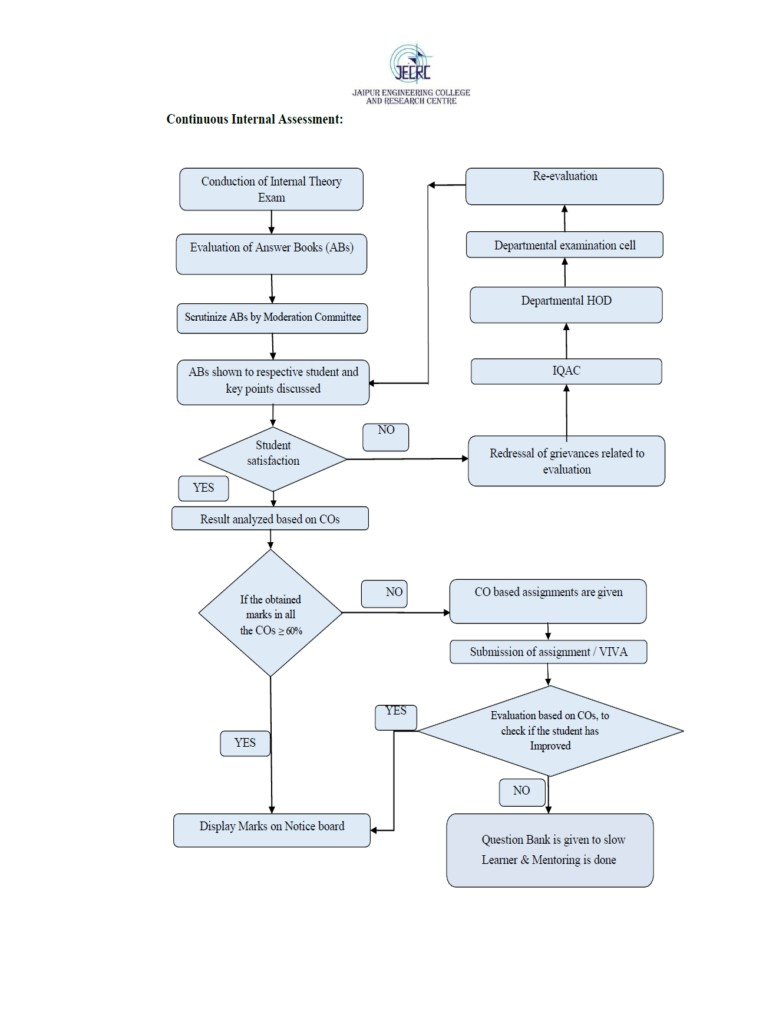JECRC Foundation

Student assessment & guidelines

##### Mechanism of internal assessment is transparent and robust in terms of frequency and mode

Question Paper Formation for Theory Exam:Process of question paper formation for internal theory exam:

1. Prior information is provided to faculty members to prepare the question paper with solution of their respective subject.

2. Then mapping of question papers to Cos will be done.

3. Then scheme of CO Wise evaluation for question paper is define.

4. In the next step Moderation Committee scrutinize all papers.

5. If at-least two papers are up to the mark, then Moderation Committee selects final question paper(s).

6. If none of the papers are up to the mark then follow the step 2 to step 6 in an iterative manner.Process of conduction of internal theory exam:

1. Once the conduction of internal theory exam i. e. midterms is over then evaluation of answer books is started.

2. In the next step Moderation Committee manages/performs the scrutinizing process of all evaluated answer books.

3. Then the answer books are shown to students, if student is satisfied with the evaluation then the result analysis based on COs will be done.

4. If the student is not satisfied with the answer book evaluation, grievances of students related to evaluation are processed through IQAC, Dept. HOD & Moderation Committee by submitting a grievance form.

5. If the obtained marks in all COs ≥ 60%, then the student is considered as strong and assignment based on COs is given to students.

6. If the obtained marks in all COs < 60%, then the student is considered as weak and assignment based on Cos, question bank is given to students. After the evaluation of assignment based on COs, marks on notice board are displayed.

7. If any students are still found weak by following the above process then mentoring of the students will be done.

8. Redressal / Grievance related to evaluation is processed by informing students to fill up form regarding same and further processed by reevaluating the Answers book and updating the marks if any changes take place.

9. Practical subjects are assessed through: For internal assessment of practical, evaluation is based on different parameters like Practical Records, Performance, and Viva-Voce. Day to day performance of the students is assessed for every experiment which includes regularity, performance, viva, and promptness in submitting the record.

© JECRC Foundation 2020